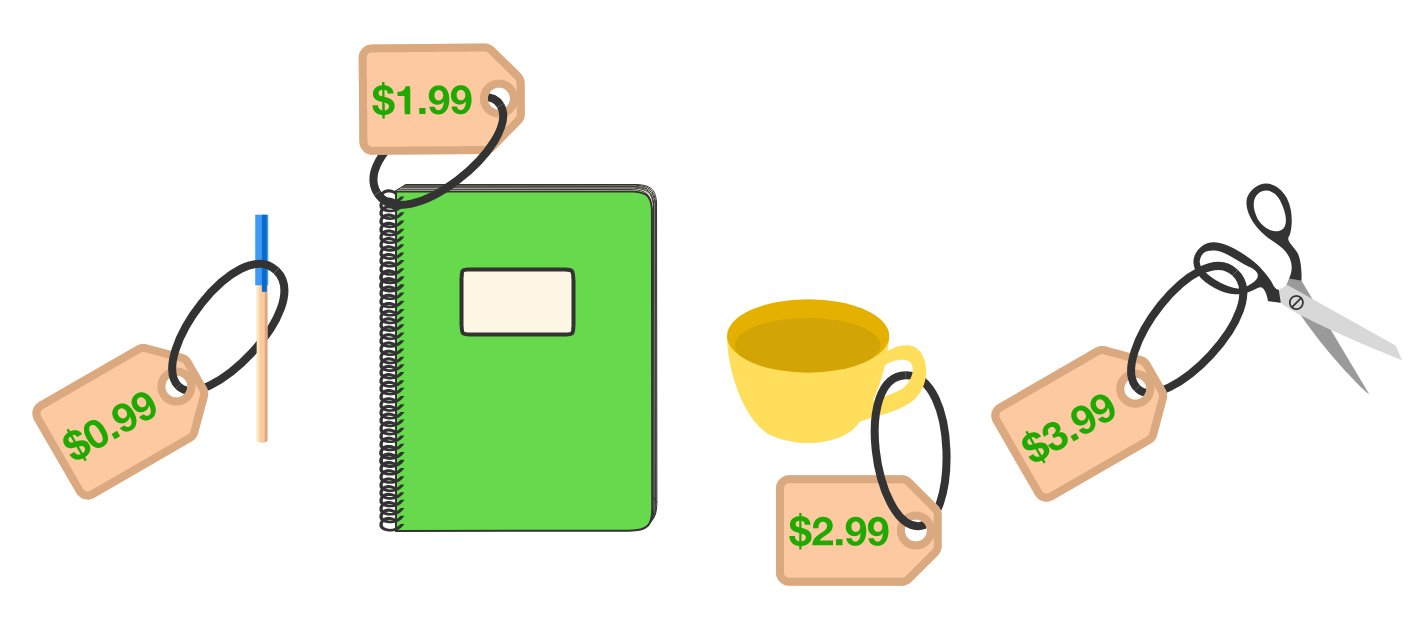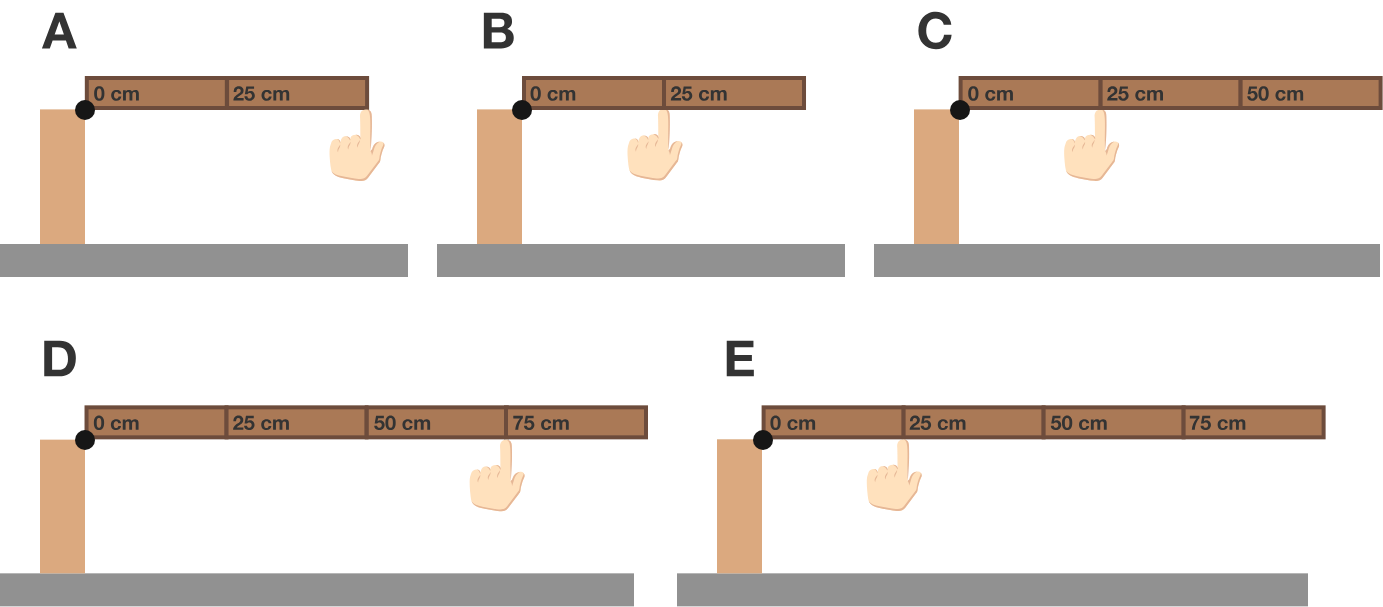# Problems of the Week

Contribute a problem

# 2018-09-03 Basic

Marie shops at a store where all prices end in 99 cents ($0.99,$1.99, $2.99, etc.). She ends up spending$33.89.

How many items did she purchase?Measuring sticks of various lengths are hinged at the edge of a table, with a fingertip supporting the stick somewhere along the stick's length.

When is the force applied by the fingertip the greatest?Details and Assumptions:

• All measuring sticks are made of the same material and have the same width and thickness.
• All measuring sticks are divided into $25 \text{ cm}$ segments.

These images show the same part of the night sky as seen in January and July 2018. Most of the stars have remained fixed, though two seem to have moved relative to the others.

Which star is closer to us, red or blue?Assume that the red and blue stars have not moved significantly relative to the Sun in the time between the two images were taken, and that the colors are used simply to label the stars (they do not indicate frequency shifts).

Out of a group of students, 60% are taking mathematics, 65% French, and 75% German, but none are taking all 3 subjects.

Is there a student who is taking neither French nor German?

$A,B,C$ are integers satisfying ${\large A^{B^{^C}} = 2^{2^{^2}}}.$ One possible solution is $(A,B,C) = (2,2,2).$

How many other solutions $(A,B,C)$ are there?

×